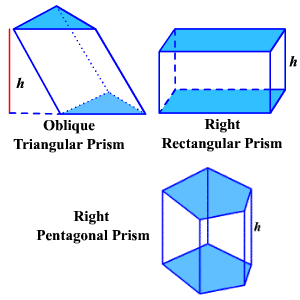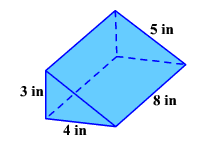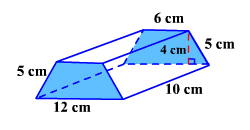Surface Area of a Prism

The lateral surface area of a prism is the sum of the areas of its lateral faces.

The total surface area of a prism is the sum of the areas of its lateral faces and its two bases.Usually, if "right" or "oblique" is not mentioned, you can assume the prism is a right prism.

The general formula for the lateral surface area of a right prism is  $L.S.A.=ph$  where  $p$ represents the perimeter of the base and $h$ represents the height of the prism.

Example 1:

Find the lateral surface area of a triangular prism with bases edges $3$ inches, $4$ inches and $5$ inches and altitude $8$ inches.The perimeter is the sum of the bases.

$p=3+4+5=12$ inches

Lateral Surface Area $=12\left(8\right)=96\text{\hspace{0.17em}}\text{\hspace{0.17em}}{\text{inches}}^{2}$

The general formula for the total surface area of a right prism is $T.S.A.=ph+2B$ where $p$ represents the perimeter of the base, $h$ the height of the prism and $B$ the area of the base.

There is no easy way to calculate the surface area of an oblique prism in general. The best way is to find the areas of the bases and the lateral faces separately and add them.

Example 2:

Find the total surface area of an isosceles trapezoidal prism with parallel edges of the base $6$ cm and $12$ cm, the legs of the base $5$ cm each, the altitude of the base $4$ cm and height of the prism $10$ cm.The perimeter of the base is the sum of the lengths of the sides.

$p=6+5+12+5=28\text{\hspace{0.17em}}\text{\hspace{0.17em}}\text{cm}$

Since the base is an isosceles trapezoid, its area is $\frac{1}{2}h\left({b}_{1}+{b}_{2}\right)$ .

$B=\frac{1}{2}\left(4\right)\left(6+12\right)=36\text{\hspace{0.17em}}{\text{cm}}^{2}$

$\begin{array}{l}T.S.A.=ph+2B\\ \text{\hspace{0.17em}}\text{\hspace{0.17em}}\text{\hspace{0.17em}}\text{\hspace{0.17em}}\text{\hspace{0.17em}}\text{\hspace{0.17em}}\text{\hspace{0.17em}}\text{\hspace{0.17em}}\text{\hspace{0.17em}}\text{\hspace{0.17em}}\text{\hspace{0.17em}}\text{\hspace{0.17em}}\text{\hspace{0.17em}}\text{\hspace{0.17em}}\text{\hspace{0.17em}}=28\left(10\right)+2\left(36\right)\\ \text{\hspace{0.17em}}\text{\hspace{0.17em}}\text{\hspace{0.17em}}\text{\hspace{0.17em}}\text{\hspace{0.17em}}\text{\hspace{0.17em}}\text{\hspace{0.17em}}\text{\hspace{0.17em}}\text{\hspace{0.17em}}\text{\hspace{0.17em}}\text{\hspace{0.17em}}\text{\hspace{0.17em}}\text{\hspace{0.17em}}\text{\hspace{0.17em}}\text{\hspace{0.17em}}=280+72=352\text{\hspace{0.17em}}\text{\hspace{0.17em}}{\text{cm}}^{2}\end{array}$